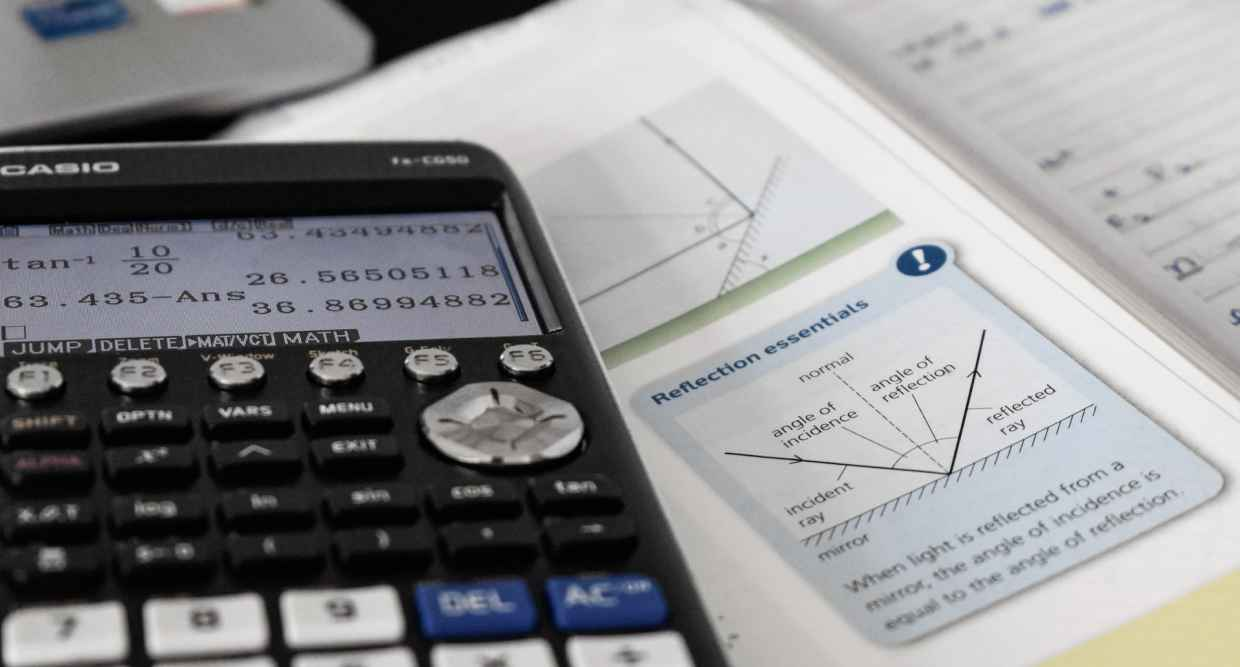Syllabus
How
CBSE 10

### How Riya Overcame Her Trigonometry Issues: 7 Essential Tips and Tricks for Class 10 CBSE SyllabusApr 17, 2023Are you struggling with trigonometry in Class 10 CBSE syllabus? Look no further! Meet Riya, a student just like you, who overcame her struggles and mastered trigonometry with these 7 essential tips and tricks. From understanding the basics to practicing word problems, Riya shares how she succeeded in trigonometry. Plus, take your learning to the next level with Aha, an AI learning tool that provides abundant practices and evaluation tools to help you ace your exams.

## 1. Understand the Basics

Riya realized that having a clear understanding of the basics of trigonometry was crucial before starting with complex problems. She learned about the three basic trigonometric ratios, sine (sin), cosine (cos), and tangent (tan), which are defined as follows:

• Sine (sin) = Opposite/Hypotenuse

By understanding these basic ratios, Riya was able to solve complex problems with ease.

## 2. Practice, Practice, Practice

Riya understood that trigonometry was all about practice. She started with simple problems and gradually moved towards the complex ones. She used online resources and practice books to sharpen her skills.

## 3. Learn the Identities

Trigonometry identities are equations that are true for all values of the variables. Riya learned about the common trigonometric identities:

• sin²θ + cos²θ = 1
• 1 + tan²θ = sec²θ
• 1 + cot²θ = csc²θ

Learning these identities made solving problems easier for her.

## 4. Draw Diagrams

Riya realized that drawing diagrams was an essential part of trigonometry. It helped her visualize the problem and find the solution. She always drew clear and accurate diagrams to avoid confusion and mistakes.

## 5. Memorize the Table of Values

Riya memorized the table of trigonometric values, which saved her a lot of time while solving problems. Here is the table of values for the trigonometric ratios:

## 6. Learn to Use Trig Functions on Your Calculator

Using a calculator to solve trigonometric problems can be very helpful. You can use the calculator's trigonometric functions to find the value of a ratio or an angle. First, make sure your calculator is set to the correct mode (degrees or radians). Then, enter the value of the ratio or angle you are trying to find. Finally, press the appropriate trigonometric function button (sin, cos, or tan) to get the answer. Remember to round your answer to the correct number of decimal places!